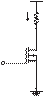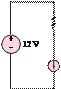### Create an Account

Already have account?

### Forgot Your Password ?

Home / Questions / Determine vGS vDS and iD for the transistor amplifier of Figure 119 in the text Assume

# Determine vGS vDS and iD for the transistor amplifier of Figure 119 in the text Assume

Determine vGS , vDS , and iD  for the transistor amplifier of Figure 11.9 in the text. Assume VDD  = 12 V; RD  = 10 ks-2R1  = R2  = 2 Ms-2RS  = 10 ks-2VT  = 1 V; K = 1 mA/V2.

11.17      The power MOSFET circuit of Figure P11.17 is configured as a voltage-controlled current source. Let = 1.5 A/V2 and V= 3 V.

a.    If V= 5 V, find the range of Rfor which the VCCS will operate.

b.    If RL  = 1 s-2, determine the range of VG  for which the VCCS will operate.2.0

1.8

1.6

1.4

1.2

1.0

12 VR

ID                   RL

L

Û

VGTA = 25°C vGS = 10 V 9 V 8 V 7 V 6 V 5 V 4 V 3 V

0.8

0.6

0.4

0.2

0

0

1.0

2.0      3.0

4.0       5.0       6.0       7.0       8.0    9.0       10

Jun 18 2020 View more View Less

#### Answer (Solved)Subscribe To Get Solution